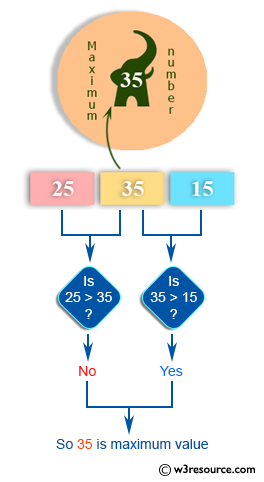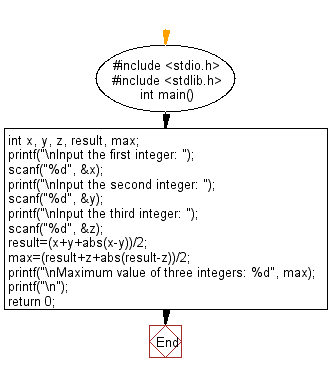﻿ C Program: Taking three integers and find the maximum

# C Exercises: Accepts three integers and find the maximum

## C Basic Declarations and Expressions: Exercise-13 with Solution

Write a C program that accepts three integers and finds the maximum of three.

Pictorial Presentation:C Code:

``````#include <stdio.h>
#include <stdlib.h>
int main()
{
int x, y, z, result, max; // Declare variables

// Prompt user for the first integer and store in 'x'
printf("\nInput the first integer: ");
scanf("%d", &x);

// Prompt user for the second integer and store in 'y'
printf("\nInput the second integer: ");
scanf("%d", &y);

// Prompt user for the third integer and store in 'z'
printf("\nInput the third integer: ");
scanf("%d", &z);

// Calculate the result
result = (x + y + abs(x - y)) / 2;

// Calculate the maximum value
max = (result + z + abs(result - z)) / 2;

// Print the maximum value
printf("\nMaximum value of three integers: %d", max);
printf("\n");

return 0;
}
``````

Sample Output:

```Input the first integer: 25

Input the second integer: 35

Input the third integer: 15

Maximum value of three integers: 35
```

Flowchart:C Programming Code Editor:

What is the difficulty level of this exercise?

Test your Programming skills with w3resource's quiz.

﻿